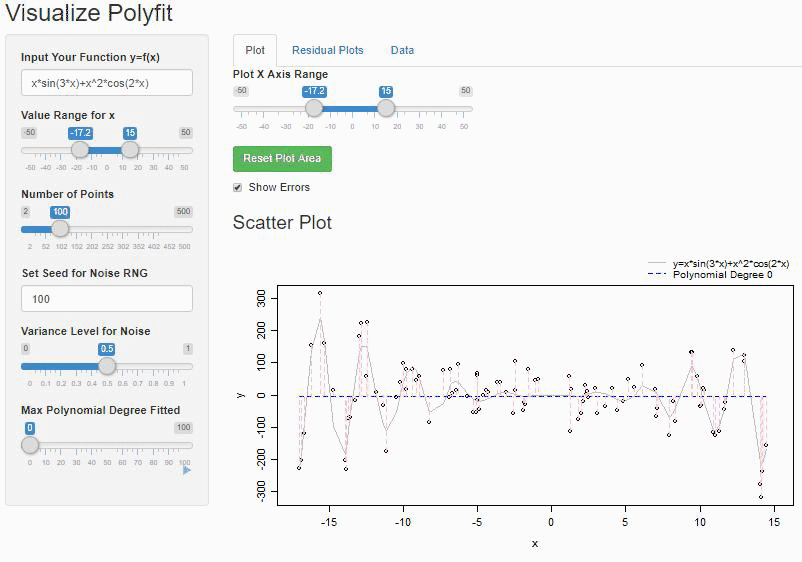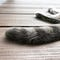# Polynomial Regression〰️http://stevenlio.shinyapps.io/polyfit

# Introduction

I am a believer in Linear Algebra and always fascinated with its powerful application. Linear Regression is one of the first applications of Linear Algebra that I’ve learned. Simply put, Linear Regression is a process of generalizing the linear relationship between a scalar variable (dependent~y) and one or more explanatory variables (independent~x) by minimalizing the distance between the data and the regression function. a.k.a. the line of best fit in Simple Linear Regression.

# Hello World! 🌎

Hello internet, I am a new user 🐣! Just trying out the medium platform, let's see how it goes! And to include some tips for me for the future 👍.A Lazy Morning — Photo by Steven Lio

# Display some of my favorite Math:

Einstein’s famous Mass-energy equivalence formula 🌌: